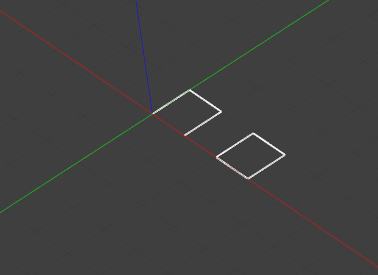## function Extrude

Extrude( (object)entities, (Vec3​)extrusion_vector, (object)make_solid, (object)assign_new_color) -> Body

Extrudes a body out of a surface.

Member of module model.

``````import s4l_v1.model as model
from s4l_v1.model import Vec3

p1 = model.CreatePolyLine([Vec3(0,0,0), Vec3(0,1,0),Vec3(1,1,0), Vec3(1,0,0)])
model.Extrude([p1],Vec3(0,0,5),True,False)

p2 = model.CreatePolyLine([Vec3(2,0,0), Vec3(2,1,0),Vec3(3,1,0), Vec3(3,0,0), Vec3(2,0,0)])
model.Extrude([p2],Vec3(0,0,5),True,False)
``````